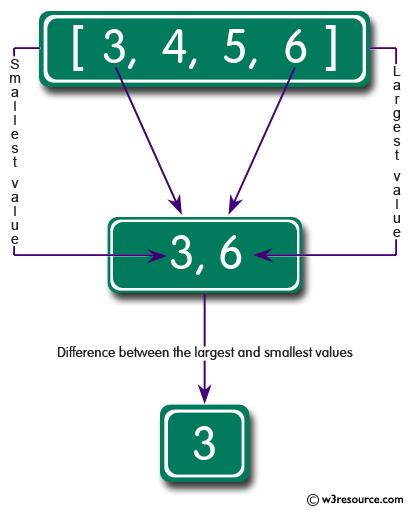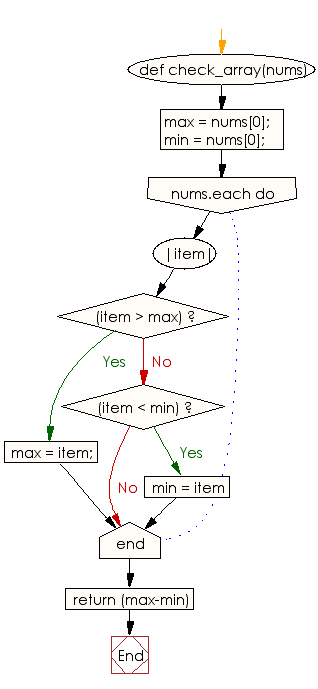﻿ Ruby Array exercises: Find the difference between the largest and smallest values of an given array of integers and length 1 or more - w3resource# Ruby Array Exercises: Find the difference between the largest and smallest values of a given array of integers of length 1 or more

## Ruby Array: Exercise-30 with Solution

Write a Ruby program to find the difference between the largest and smallest values of a given array of integers of length 1 or more.Ruby Code:

``````def check_array(nums)
max = nums;
min = nums;
nums.each do |item|
if(item > max)
max = item;
elsif(item < min)
min = item
end
end
return (max-min)
end

print check_array([3, 4, 5, 6]),"\n"
print check_array([3, 4, 5]),"\n"
print check_array([3, 4])
``````

Output:

```3
2
1
```

Flowchart:Ruby Code Editor: